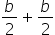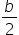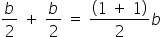Mathematics
Easy

Question

# Write an equivalent expression for the expression.

## b    2b    4bHint:

## The correct answer is: b

### The given expression isThere is one variable in the above expression. The variable is: ‘b’. There are two terms in the above expression. We have to perform the operations between them and simplify the expression.If we see, both the term contains the same variable. Such terms are called “like terms.” And the terms with different variables are called “unlike terms.For like terms, we can perform the operations between their coefficients by keeping the variable constant. It means we can treat each term as a constant and perform operations like normal operations on constants.For the above expression, we can perform an operation between the first term and second term as both the terms as they have the same variable ‘b’. We will keep ‘b’ constant and perform addition between the coefficients.The given expression has same denominator, so we can add the numerators of the expression.. .Therefore, the value of the equation is bSo option which is ‘b’, is the right option.

We have to see if the variables are same or different before performing operations. If variables are same, they operate with each other.

### Related Questions to study#### With Turito Foundation.#### Get an Expert Advice From Turito.